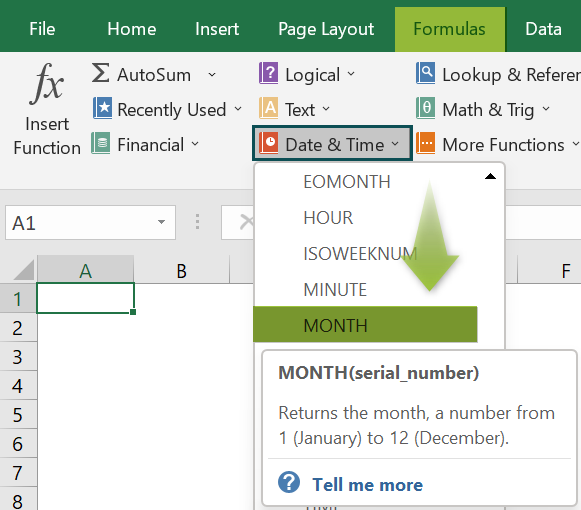# MONTH Excel FunctionArticle byExcelMojo Team## What Is MONTH Function In Excel?

The MONTH Function in Excel returns the month part from the selected date. Since there are 12 months in a year, the return value will always be an integer between 1 and 12. The MONTH Excel function is a “Date & Time” inbuilt function, so we can insert the formula from the “Function Library” or enter it directly in the cell.

For example, we will calculate the month value using the MONTH Excel function for the given date.

Select cell B2, enter the formula =MONTH(A2), and press “Enter”.

The result is ‘10’, as shown above.

###### Key Takeaways
• The MONTH Excel function returns the month value of the given date, irrespective of the date format, whether long date or short date,
• The result of the function will always be in-between the integers 1 to 12, as there are 12 months in a calendar year.
• We can find the months name instead of the month value using the TEXT and DATE functions along with the valid month syntax like “mm”, “mmm”, “mmmm”, etc.
• We can convert the month name to the month value using the DATEVALUE function.

### MONTH() Excel Formula

The syntax of the MONTH Excel Formula is,

The argument of the MONTH Excel Formula is,

• serial_number: It is a mandatory argument. It is a valid Excel date to get the month value.

### How To Use MONTH Excel Function?

We can use the MONTH Excel function in 2 ways, namely,

1. Access from the Excel ribbon.
2. Enter in the worksheet manually.

#### Method #1 – Access from the Excel ribbon

Choose an empty cell for the result → select the “Formulas” tab → go to the “Function Library” group → click the “Date & Time” option drop-down → select the “MONTH” function, as shown below.

The “Function Arguments” window appears. Enter the argument value in the “Serial_number” field → click “OK”, as shown below.

#### Method #2 – Enter in the worksheet manually

1. Select an empty cell for the output.
2. Type =MONTH ( in the selected cell. [Alternatively, type =M and double-click the MONTH function from the list of suggestions shown by Excel.]
3. Enter the argument as the cell value or cell reference in Excel and close the brackets.
4. Press the “Enter” key.

We will calculate the month value using MONTH Excel function for the dates in different formats.

In the table, the data is,

• Column A contains the Date.
• Column B displays the Output.

The steps to find the month using MONTH Excel Function is,

1. Select cell B2, enter the formula =MONTH(A2), and press the “Enter” key. The result is “1”, as shown below.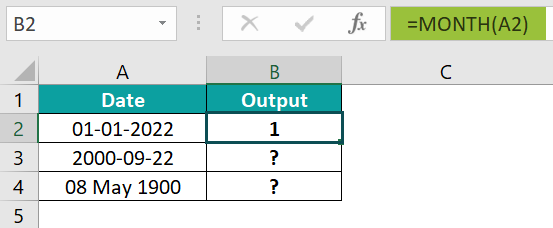2. Drag the formula from cell B2 to B4 using the fill handle.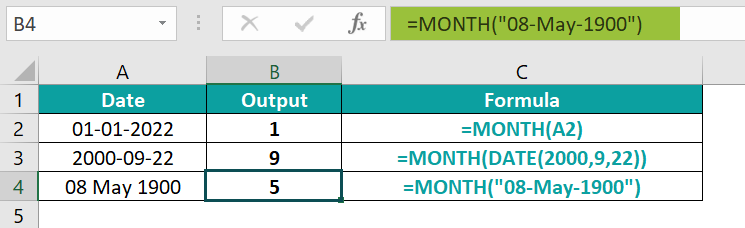We get the output shown above. Column C is for our reference, for the formulas that can be used in place of dragging the formula.

### Examples

We will understand some advanced scenarios with MONTH Excel Function examples.

#### Example #1

We will find the month name instead of the month number/value using the Excel TEXT function for dates in different formats.

In the table, the data is,

• Column A contains the Date.
• Column B displays the Output.

The steps to evaluate the dates by the TEXT Function in Excel are,

• Step 1: Select cell B2, enter the TEXT formula =TEXT(A2,“mmmm”), and press the “Enter” key. The result is “June”, as shown below.
• Step 2: Select cell B3, enter the TEXT formula =TEXT(A3,”mmm”), and press the “Enter” key. The result is “Dec”, as shown below.

We get the output shown above. Column C is for our reference.

#### Example #2

We will find the month name instead of the month number/value using the Excel TEXT function for dates in different formats.

In the table, the data is,

• Column A contains the Date.
• Column B displays the Output.

The procedure to evaluate the dates by the TEXT Function in Excel is,

Enter the formulas as follows:

• =TEXT(DATE(1989,12,1),“mmm”) in cell B2,
• =TEXT(DATE(1999,5,2),”mmmm”) in cell B3,
• =TEXT(DATE(2009,7,3),”mmmmm”)in cell B4, and
•  Press the “Enter” key after every formula entry.

We get the output shown above. Column C is for our reference.

#### Example #3

We will convert the month name into the month number using the MONTH function with the DATEVALUE Excel function depicting the month name.

In the table, the data is,

• Column A contains the Month Name.
• Column B displays the Month Number as output.

The steps to find the month value using the MONTH and DATEVALUE functions are,

• Step 1: Select cell B2, enter the formula =MONTH(DATEVALUE(A2 & “1”)), and press the “Enter” key. The result is “1”, as shown below.
• Step 2: Drag the formula from cell B2 to B13 using the fill handle. We get the output shown below.

#### Example #4

We will calculate the month value using the MONTH Excel function for the dates.

In the table, the data is,

• Column A contains the Date.
• Column B displays the Output.

The steps to evaluate the dates using the MONTH Function in Excel are,

• Step 1: Select cell B2, enter the formula =MONTH(A2), and press the “Enter” key. The result is “12”, as shown below.
• Step 2: Drag the formula from cell B2 to B13 using the fill handle. We get the output shown below.

### Important Things To Note

• The #VALUE! error occurs,
• When the function doesn’t recognize the date format, it may be an invalid format.
• If the entered date value is earlier than 1 Jan 1900.
• If the date entered is invalid, such as the month is greater than 12, or if the given date is greater than 30 or 31 in that particular month.
• No number must be entered as an argument. If entered, the result will, by default, be 1. For ex, =MONTH(15), =MONTH(2), all will return 1 as output.

1. What does the MONTH function do in Excel?

The MONTH Function in Excel is used to find the month for a valid date format.

The syntax of the MONTH function is =MONTH(serial_number).

2. How does the MONTH function work in Excel?

The MONTH function works in Excel by following the below steps;

1) Select an empty cell for the output.
2) Type =MONTH ( in the selected cell. [Alternatively, type =M and double-click the MONTH function from the list of suggestions shown by Excel.]
3) Enter the argument as the cell value or cell reference and close the brackets.
4) Press the “Enter” key.

For example, we will calculate the month value using the MONTH Excel function.
In the table, the data is,
Column A contains the Date.
Column B displays the Output.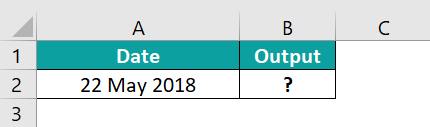The procedure to evaluate the dates by the MONTH Function in Excel is,

Select cell B2, enter the formula =MONTH(A2), and press the “Enter” key. The result is 5, as shown below.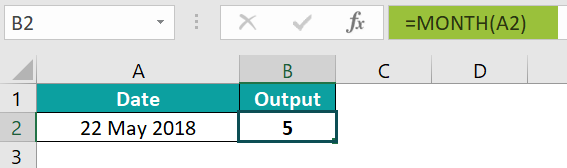3. Where is the MONTH Function in Excel?

One can activate the MONTH Function in Excel using the following steps:

Choose an empty cell for the result → select the “Formulas” tab → go to the “Function Library” group → click the “Date & Time” option drop-down → select the “MONTH” function, as shown below.